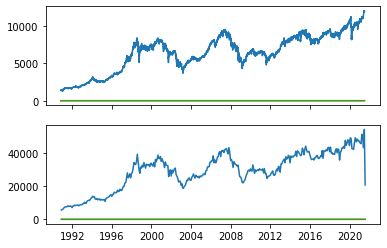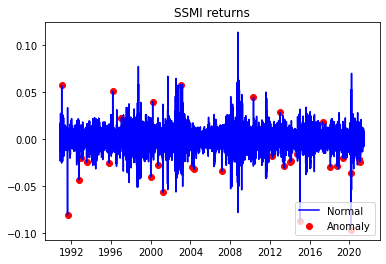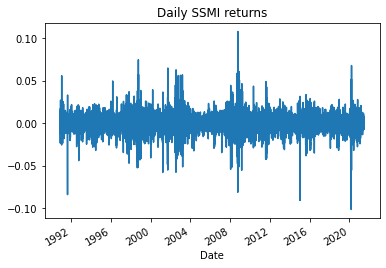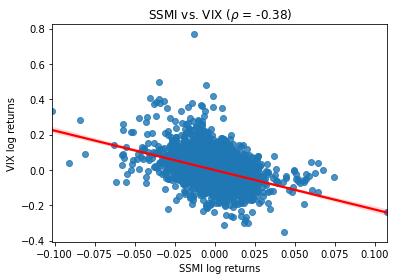# Switzerland(SMI) stock market

## What is the name of Switzerland's (SMI) stock market?

The SMI is a symbol of the Switzerland(SMI) stock market.
The Swiss Market Index (SMI), often referred to as the SMI, is the most prominent stock market index in Switzerland. It is the benchmark index for the Swiss equity market, representing the performance of the largest and most liquid publicly traded companies listed on the SIX Swiss Exchange, which is the principal stock exchange in Switzerland. Here are some key details about the Swiss Market Index (SMI):

Composition: The SMI is composed of the 20 largest and most actively traded companies listed on the SIX Swiss Exchange. These companies come from various sectors, including finance, pharmaceuticals, healthcare, technology, and consumer goods. The composition of the index is reviewed and adjusted regularly to ensure it reflects the current market conditions.

Market Capitalization Weighted: The SMI is a market capitalization-weighted index. This means that the weight of each component stock in the index is determined by its market capitalization, which is the product of its stock price and the number of outstanding shares. Larger companies have a more significant impact on the index's performance.

Diversification: The SMI offers diversification across different sectors of the Swiss economy, reducing concentration risk. Key industries represented in the index include banking and financial services (e.g., Credit Suisse, UBS), pharmaceuticals (e.g., Roche, Novartis), food and beverages (e.g., Nestlé), and industrial companies.

Global Significance: While the SMI primarily reflects the performance of Swiss-listed companies, it is closely monitored by investors and financial professionals worldwide. Switzerland's financial market stability, strong economy, and the global reach of Swiss corporations contribute to the index's international significance.

## How to get Switzerland(SMI) stock market data?

you get data from Yahoo Finance, Quanda, google, etc.

### How to install data in Python?

Here install python lib.
import yfinance as yf
import numpy as np
import pandas as pd
import seaborn as sns
import scipy.stats as scs
import statsmodels.api as sm
import statsmodels.tsa.api as smt
import matplotlib.pyplot as plt

#### Some Python code data collected from Yahoo Finance

start='1985-01-01',
end='2021-07-07',
progress=False)
How to get individual column SMI adj price?

#### Simple return and Log return SSMI

df[['simple_rtn','log_rtn']].tail(20)

#### How to get realized volatility SMI in Python?

def realized_volatility(x):
return np.sqrt(np.sum(x**2))
df_rv = df.groupby(pd.Grouper(freq='M')).apply(realized_volatility)
df_rv.rename(columns={'log_rtn''rv'}, inplace=True)
df_rv.rv = df_rv.rv * np.sqrt(12)
fig, ax = plt.subplots(21, sharex=True)
ax.plot(df)
ax.plot(df_rv)
Plot Realised volatility SMI
[<matplotlib.lines.Line2D at 0x7f8af4356f50>,
<matplotlib.lines.Line2D at 0x7f8af4366090>,
<matplotlib.lines.Line2D at 0x7f8af4366250>]Plot time series
fig, ax = plt.subplots(31, figsize=(2420), sharex=True)
ylabel = 'Stock price ($)') df.simple_rtn.plot(ax=ax) ax.set(ylabel = 'Simple returns (%)') df.log_rtn.plot(ax=ax) ax.set(xlabel = 'Date', ylabel = 'Log returns (%)') #### Get mean and standard deviation df_rolling = df[['simple_rtn']].rolling(window=21) \ .agg(['mean''std']) df_rolling.columns = df_rolling.columns.droplevel() df_outliers = df.join(df_rolling) def indentify_outliers(rown_sigmas=3): x = row['simple_rtn'] mu = row['mean'] sigma = row['std'] if (x > mu + 3 * sigma) | (x < mu - 3 * sigma): return 1 else: return 0 #### How do plot Outliers? df_outliers['outlier'] = df_outliers.apply(indentify_outliers, axis=1) outliers = df_outliers.loc[df_outliers['outlier'] == 1, ['simple_rtn']] fig, ax = plt.subplots() ax.plot(df_outliers.index, df_outliers.simple_rtn, color='blue', label='Normal') ax.scatter(outliers.index, outliers.simple_rtn, color='red', label='Anomaly') ax.set_title("SSMI returns") ax.legend(loc='lower right') <matplotlib.legend.Legend at 0x7f8aef0d5690>r_range = np.linspace(min(df.log_rtn), max(df.log_rtn), num=1000) mu = df.log_rtn.mean() sigma = df.log_rtn.std() norm_pdf = scs.norm.pdf(r_range, loc=mu, scale=sigma) fig, ax = plt.subplots(12, figsize=(168)) # histogram sns.distplot(df.log_rtn, kde=False, norm_hist=True, ax=ax) ax.set_title('Distribution of SSMI returns', fontsize=16) ax.plot(r_range, norm_pdf, 'g', lw=2, label=f'N({mu:.2f}{sigma**2:.4f})') ax.legend(loc='upper left'); df.log_rtn.plot(title='Daily SSMI returns') <matplotlib.axes._subplots.AxesSubplot at 0x7f8aeeea6810>How to compare SSMI and VIX? df = yf.download(['^SSMI''^VIX'], start='1985-01-01', end='2021-07-07', progress=False) ax = sns.regplot(x='log_rtn', y='vol_rtn', data=df, line_kws={'color''red'}) ax.set(title=f'SSMI vs. VIX ($\\rho$= {corr_coeff:.2f})', ylabel='VIX log returns', xlabel='SSMI log returns') [Text(0, 0.5, 'VIX log returns'), Text(0.5, 0, 'SSMI log returns'), Text(0.5, 1.0, 'SSMI vs. VIX ($\\rho\$ = -0.38)')]